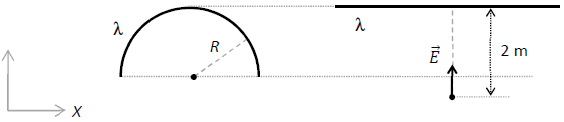# Find the linear charge density lambda and the radius of a charged semicircle

Homework Statement:
A uniformly loaded semicircle-shaped wire of radius R has a linear density of charge lambda. An electric field magnitude of 10 N / C is measured at the center of the semi-circle. We straighten this same wire and we note an electric field magnitude of 8 N / C, this time at a distance of 2 m on its perpendicular bisector; the field thus measured is directed towards the wire.

a) Calculate the values of the lambda and R
b) How many electrons would need to be added or removed from the wire in order for it to become neutral? Specify whether to add or remove electrons
c) Calculate the vector electric force on a proton placed at the center of curvature of the wire
when the latter is in the shape of a semicircle
Relevant Equations:
At the center of the semi-circle an electric field magnitude of 10 N / C
Straighten wire has an electric field magnitude of 8 N / CElectric field for the semi-circle

$$E = - \frac {πKλ} {2R}$$

In this case E is equals to 10 N/C

Electric field for the straighten wire

$$E = 2Kλ * ( 1 - \frac {2y} {\sqrt{4y^2 + L^2}})$$

In this case E is equals to 8 N/C

What I'm searching is R, λ, and the length of the wire, so I think I may need a third equation ?

Not sure if I took the right approach for this.

Thank you.

Last edited by a moderator:

## Answers and Replies

haruspex
Science Advisor
Homework Helper
Gold Member
2020 Award
what is the relationship between L and R?

L is equal to 2 * π * R ?

haruspex
Science Advisor
Homework Helper
Gold Member
2020 Award
L is equal to 2 * π * R ?
Right, so substituting that you have two equations and two unknowns, yes?

That's right, so I can do:

$$E = - \frac {πKλ} {2R}$$

$$E = 2Kλ * ( 1 - \frac {2y} {\sqrt{4y^2 + L^2}})$$

$$L = 2 * π * R$$

Which should give me: R = 1.18962 L = 7.4746 Lambda: 8.41483E-10

Now I can pass to the next questions. Thank you :) !